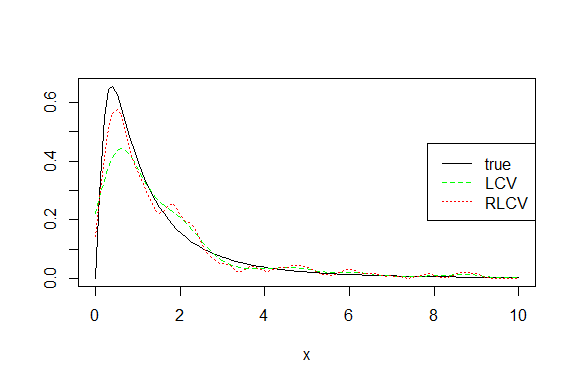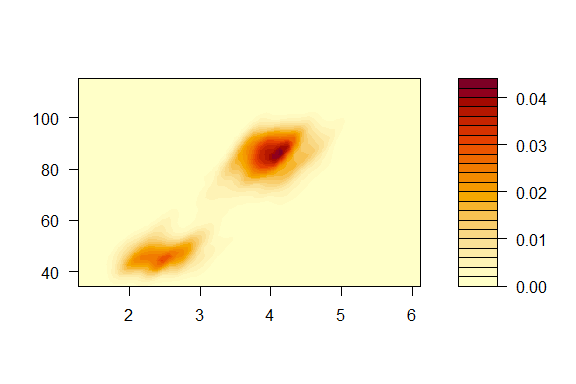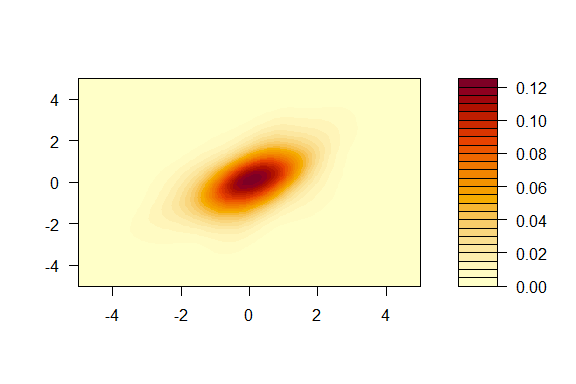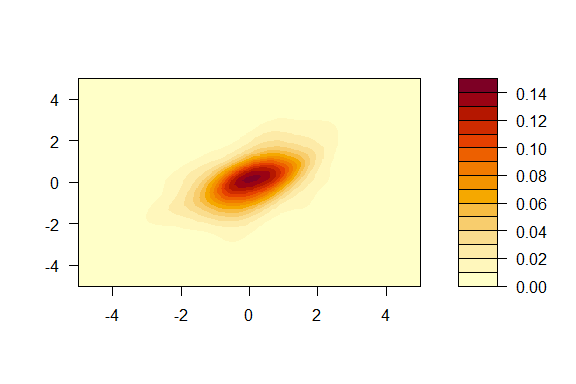# Robust Likelihood Cross Validation with rlcp

#### 2022-03-02

Likelihood Cross Valation (LCV) is commonly used to select the bandwidth in kernel density estimations. It is, however, known to be sensitive to fat-tailed distributions and/or outliers. Wu (2019) proposed a Robust Likelihood Cross Validation (RLCV) method that is resistant against fat-tailedness and/or extreme observations.

This method replaces the log-likelihood function in the cross validation objective function with a linear approximation for densities smaller than a certain threshold. It can be interpreted as a combination of LCV (for non-extreme density values, which account for the bulk of the sample) and least squares cross validation (for a few small extreme density values, if they exist in the sample). An automatic thresholding rule, depending on the sample size, number of dimension and sample covariance, has been proposed. This makes this bandwidth-selector free of tuning parameters.

The R package rlcv implements this method for uni- and multi-variate kernel density estimation. Below we illustrate the RLCV bandwidth selector with a few examples. We start with a univariate log-normal distribution. This distribution has an extended right tail. The commands for univarite LCV and RLCV are respectively lcv and rlcv. As is discussed in Wu (2019), LCV tends to oversmooth in the presence of long-tails. The comparison of these two bandwidths is consistent with the theoretical projection.

library(rlcv)
set.seed(12345)
x=rlnorm(300)
fit1=lcv(x.obs=x)
fit2=rlcv(x.obs=x)
c(fit1$h,fit2$h)
#>  0.3807475 0.1546201

Let’s examine the goodness-of-fit numerically and visually. LCV misses the peak badly, while RLCV appears to be a bit rugged. At the same time, RLCV clearly has a smaller mse.

x.new=seq(0,10,length=100)
f0=dlnorm(x.new)
f1=kde(x.obs=x,x.new=x.new,h=fit1$h) f2=kde(x.obs=x,x.new=x.new,h=fit2$h)
# compare mean squared error
mean((f0-f1)^2)
#>  0.003178948
mean((f0-f2)^2)
#>  0.001165473
# density plots
matplot(x.new,cbind(f0,f1,f2),col=c("black","green","red"),type='l',xlab='x',ylab='')
legend('right',legend = c('true','LCV','RLCV'), col = c('black','green','red'), lty = 1:3, lwd = 1 , xpd = T )Let’s move on to multivariate densities. The corresponding commands are lcv_d and rlcv_d. Consider first the old-faithful geyser data. It turns out these two methods yield almost identical bandwidths. Not surprisingly, their contour plots are nearly identical. Note that the joint distribution of the geyser data doesn’t have fat tails or outliers. This experiment confirms that LCV and RLCV produce similar results in the absence of fat-tails or outliers. This is desirable as LCV is known to be efficient (but not robust).

x=datasets::faithful
x=cbind(x[,1],x[,2])
fit1=lcv_d(x.obs=x)
fit2=rlcv_d(x.obs=x)
fit1$h #>  0.2891278 0.1665945 fit2$h
#>  0.2891273 0.1665954
x1=seq(min(x[,1])*.8,max(x[,1])*1.2,length=50)
x2=seq(min(x[,2])*.8,max(x[,2])*1.2,length=50)
x11=rep(x1,each=50)
x22=rep(x2,50)
f1=kde_d(x.new=cbind(x11,x22),x.obs=x,h=fit1$h) f2=kde_d(x.new=cbind(x11,x22),x.obs=x,h=fit2$h)
filled.contour(x1,x2,matrix(f1,50,50))filled.contour(x1,x2,matrix(f2,50,50))Lastly we look into a fat-tailed multivariate distribution. We construct a bivariate distribution by combining two univariate t distributions with five degrees of freedom via a t copula with five degrees of freedom. Again with a fat-tailed underlying distribution, LCV bandwidths tend to be larger. Although their contours look similar in shape, examination of the mse suggests that RLCV fits the true density better. The comparsion of their summary statistics also reveals that LCV oversmoothes, yielding a large gap between the true mode and fitted mode.

library(copula)
set.seed(12345)
ncop=tCopula(.5,df=5)
n=500
u=rCopula(n,ncop)
x1=qt(u[,1],5)
x2=qt(u[,2],5)
x=cbind(x1,x2)
fit1=lcv_d(x.obs=x)
fit2=rlcv_d(x.obs=x)
fit1$h #>  0.5186154 0.4273611 fit2$h
#>  0.3637373 0.3632867
# evaluation data
x1=x2=seq(-5,5,length=50)
x11=rep(x1,each=50)
x22=rep(x2,50)
f1=kde_d(x.new=cbind(x11,x22),x.obs=x,,h=fit1$h) f2=kde_d(x.new=cbind(x11,x22),x.obs=x,,h=fit2$h)
f0=dCopula(cbind(pt(x11,5),pt(x22,5)),ncop)*dt(x11,5)*dt(x22,5)
# Mean squared errors
mean((f0-f1)^2)
#>  3.889088e-05
mean((f0-f2)^2)
#>  1.959031e-05
# check summary statistics
summary(cbind(f0,f1,f2))
#>        f0                  f1                  f2
#>  Min.   :4.330e-06   Min.   :0.0000000   Min.   :0.000e+00
#>  1st Qu.:2.019e-04   1st Qu.:0.0001575   1st Qu.:3.842e-05
#>  Median :7.309e-04   Median :0.0012354   Median :1.071e-03
#>  Mean   :9.540e-03   Mean   :0.0095453   Mean   :9.551e-03
#>  3rd Qu.:4.385e-03   3rd Qu.:0.0068628   3rd Qu.:6.122e-03
#>  Max.   :1.820e-01   Max.   :0.1243200   Max.   :1.434e-01
# plot the fitted densities
filled.contour(x1,x2,matrix(f1,50,50))filled.contour(x1,x2,matrix(f2,50,50))Reference

Wu, Ximing (2019), “Robust Likelihood Cross Validation for Kernel Density Estimation,” Journal of Business and Economic Statistics, 37(4): 761-770.